# Using Excel reference functions in Pandas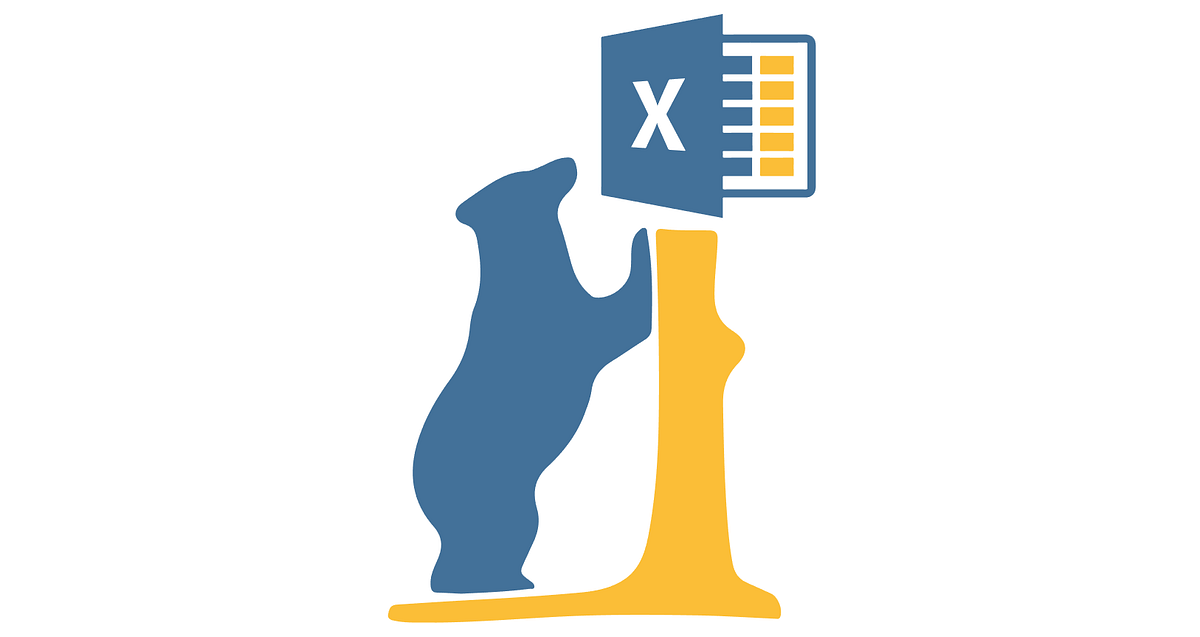Full code available at my repo. In this article, I will create a dataset from scratch using Pandas, but referencing the values of other cells while filling the dataset.

In this article, I will create a dataset from scratch using Pandas, but referencing the values of other cells while filling the dataset.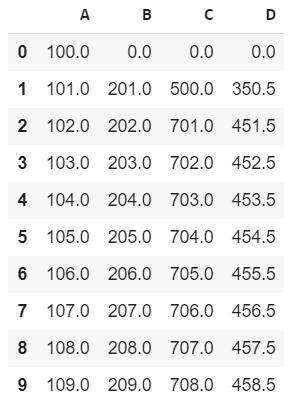I created this dataset from scratch using Pandas

One of the missing features of Pandas is creating similar functions as Excel. Excel is a great tool when we have to deal with a limited amount of information manually. One of the most intuitive features of this spreadsheet are functions.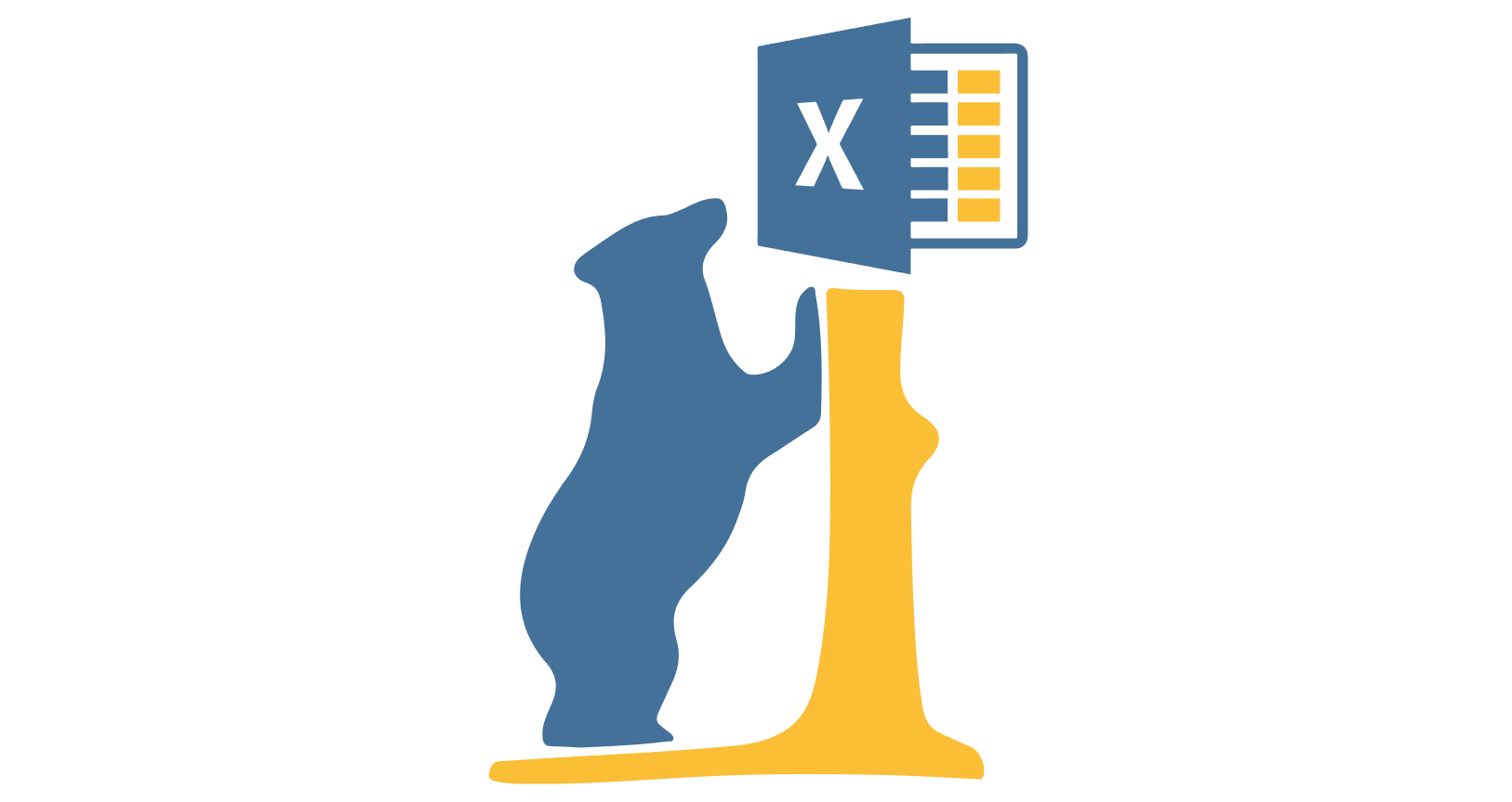how Pandas/Excel mixed Logo may look

In regards to Pandas, one of the most uncomfortable reasons why people struggle to appreciate it from the beginning is that editing data inside the dataset is really challenging. What if I want to create a dataset from scratch and I need to use functions that take info from previous rows and columns?

# Excel Version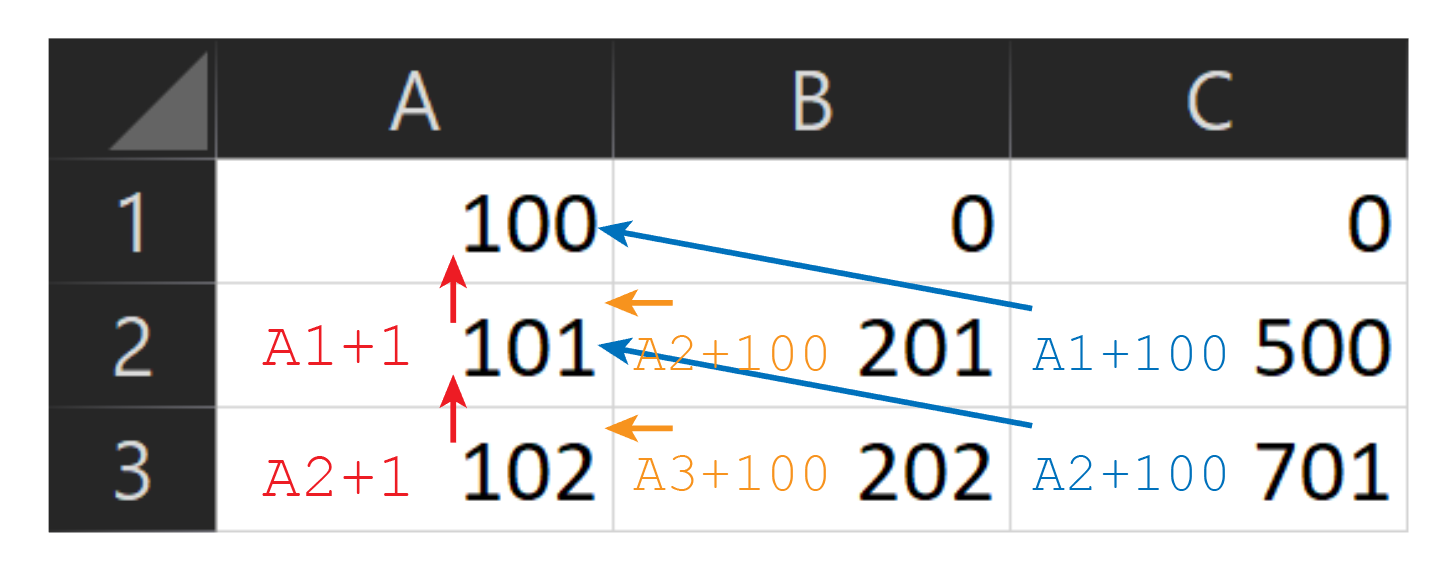Using Excel Formulas

We obtain the following dataset: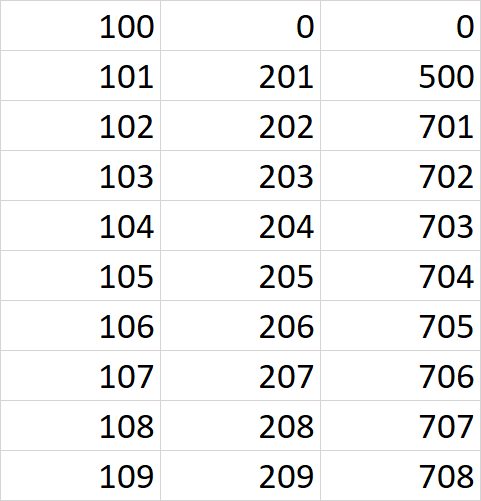10 rows of the dataset

# Pandas Version

We could save this file as a .csv, however, the purpose of this article is to replicate the same procedure with pandas. I am going to create a dataset from scratch using these relative functions.

## Creating the first row

I will be using a for the statement to create the rest of the rows. Like in Excel, the first row should not contain any function, but only data. I am just going to create an empty row to set the columns of the dataset.

``````import pandas as pd
df = [0, 0, 0]
df = pd.DataFrame(df).transpose()
df.columns = ['A', 'B', 'C']
df``````

Image for post

empty dataset

## Setting values

I could have done this before, but I prefer to proceed one step at a time.

``````df['A'] = 100
d``````

## Python Pandas Objects - Pandas Series and Pandas Dataframe

In this post, we will learn about pandas’ data structures/objects. Pandas provide two type of data structures:- ### Pandas Series Pandas Series is a one dimensional indexed data, which can hold datatypes like integer, string, boolean, float...

## Understanding Groupby() Function in Pandas Dataframe — Part 1

The groupby() function is one of the most useful functions when dealing with large dataframes in Pandas. A groupby operation typically involves a combination of splitting the object.

## Python Pandas Tutorial (Part 6): Add/Remove Columns From DataFrames

In this video, we will be learning how to add and remove our rows and columns. This video is sponsored by Brilliant. Go to https://brilliant.org/cms to sign ...

## PYTHON Pandas Basic Functions

PYTHON Pandas Basic Functions. So far, we have learned the three pandas data structure and how to create them. Due to its importance in real-time data processing, we will focus on dataframe objects right now and mention a few other data structures.

## Python Pandas: How To Add Rows In DataFrame

Python Pandas dataframe append() is an inbuilt function that is used to add rows in the dataframe. The loc[] and iloc[] is also way to add or modify rows.